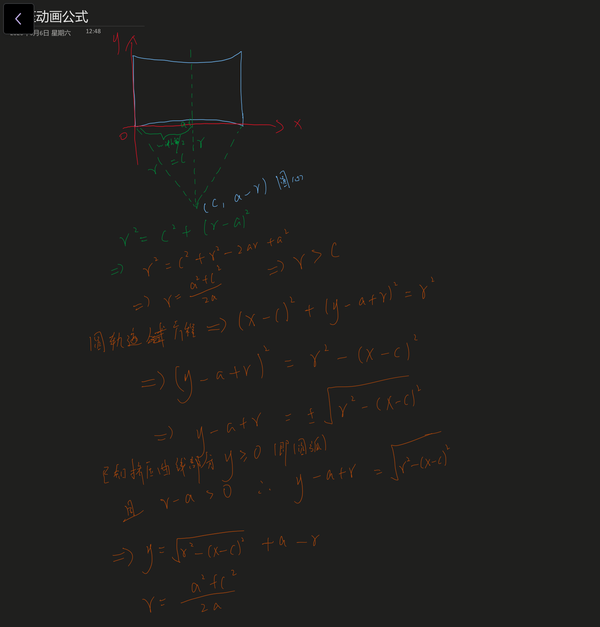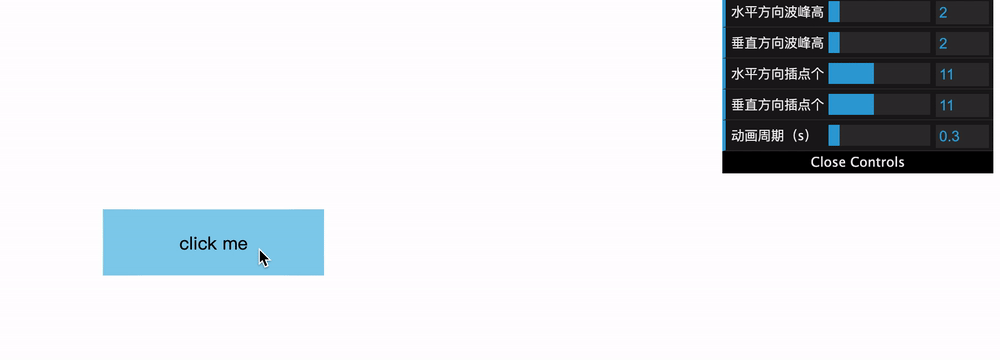## 前言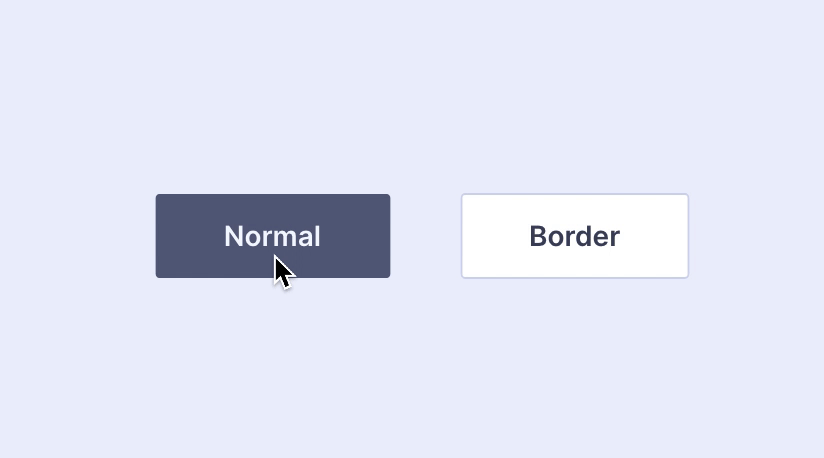• 方法1：尝试利用clip-path + animation来实现
• 方法2：尝试利用clip-path + SVG clipPath animation
• 方法3：尝试利用transformmatrix()进行矩阵变换 + animation

## 尝试

### 方法一：clip-path + animation

clip-path属性用于设置裁剪区域，使得元素只有裁剪区域的部分才会显示，最关键的是clip-path支持动画！但是经过一番尝试，clip-path目前支持的裁剪形状并不能满足挤压动画的需求，即凹曲线；目前clip-path支持的形状有：

• inset()：矩形；
• circle()：圆形；
• ellipse()：椭圆；
• polygon()：多边形；
• url()：引用SVG形状；
• 几何框盒；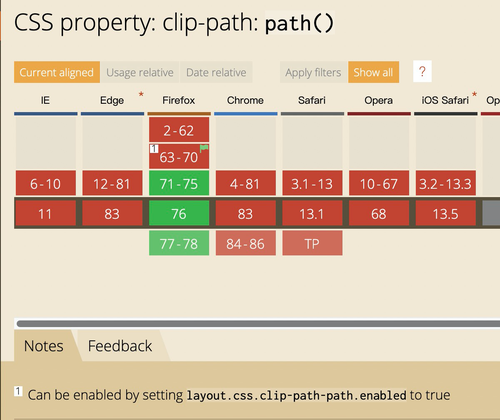### 方法二：clip-path + SVG clipPath aimation

clipPath元素内定义的形状就是裁剪区域，除了可以使用path，还可以使用SVG内其他用来定义形状的元素，如：<rect><circle>等；不仅如此，还可以使用SVG animation语法，对形状进行动画处理；但是经实践，pathd属性开启动画后，被引用时并没有预想中那样有插值关键帧过渡的效果，而是直接跳到最后一帧，也就是说clipPath内的动画对于path没有效果：

### 方法三：matrix()/matrix3d()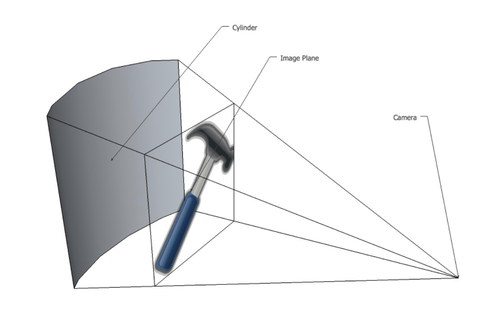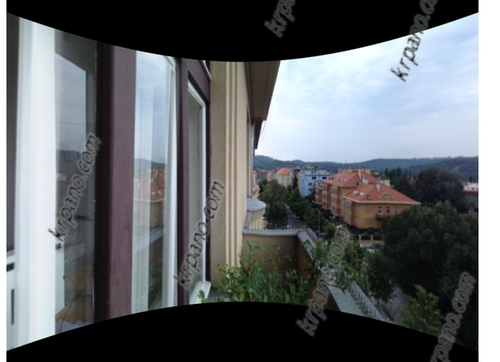## 改变思路：思考原理

### 挤压曲线的插值点坐标求解\begin{aligned}r^2 &= (r - a)^2 + c^2 \\[1em] \Rightarrow r &= \frac{a^2 + c^2}{2a} \end{aligned}

$(x - c)^2 + (y - a + r)^2 = r^2$

$y - a + r = \pm \sqrt{r^2 - (x - c)^2} \\[1em] \because y \geqslant 0 \quad \land \quad r - a > 0 \\[1em] \therefore y - a + r = \sqrt{r^2 - (x - c)^2} \\[1em] \\[1em] \Rightarrow y = \sqrt{r^2 - (x - c)^2} + a - r$

### 插值化处理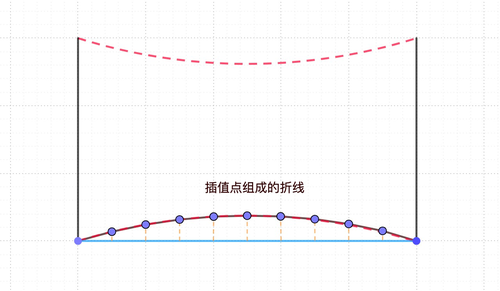## 后话